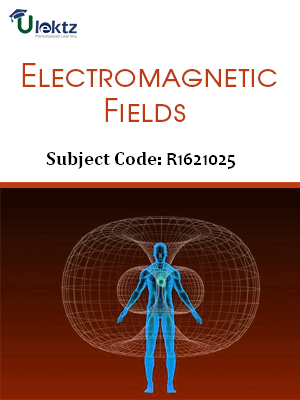•My WalletMy Order
•My Profile
•My Connections
•My Books
•My Videos
•My Tests
•My Calender
•My Messages
•My Shopping Cart
•My Orders
•Account Settings
•Help

# Book Details# Electromagnetic Fields

 Course Code : R1621025 Author : Ulektz University : JNTU Kakinada Regulation : 2016 Categories : Electronics & Communication Format :ePUB3 (DRM Protected) Type : eBook

FREE

Description :Electromagnetic Fields of R1621025 covers the latest syllabus prescribed by JNTU Kakinada for regulation 2016. Author: Ulektz, Published by uLektz Learning Solutions Private Limited.

Note : No printed book. Only ebook. Access eBook using uLektz apps for Android, iOS and Windows Desktop PC.

##### Topics
###### UNIT - I ELECTROSTATICS

1.1 Electrostatic Fields - Coulomb’s law

1.2 Electric Field Intensity (EFI) - EFI due to a line and a surface charge - Work done in moving a point charge in an electrostatic field

1.3 Electric potential - Properties of potential function - Potential gradient

1.4 Gauss law - Maxwell’s first law, div ( D )=ρv - Laplace’s and Poisson’s equations and solution of Laplace’s equation in one variable

###### UNIT - II CONDUCTORS – DIELECTRICS AND CAPACITANCE

2.1 Electric dipole – Dipole moment - Potential and EFI due to an electric dipole

2.2 Torque on an electric dipole in an electric field - Behaviour of conductors and insulators in an electric field - Polarization

2.3 Boundary conditions between conduction to dielectric and dielectric to dielectric capacitance - Capacitance of parallel plates, spherical and coaxial cables with composite dielectrics

2.4 Energy stored and energy density in a static electric field - Current density -Conduction and convection current densities

2.5 Ohm’s law in point form - Equation of continuity

###### UNIT - III MAGNETO STATICS AND AMPERE’S LAW

3.1 Static magnetic fields - Biot-Savart law - Oersted experiment

3.2 Magnetic field intensity (MFI) - MFI due to a straight current carrying filament - MFI due to circular, square and solenoid current carrying wire

3.3 Relation between magnetic flux, magnetic flux density and MFI - Maxwell’s second Equation, div(B)=0

3.4 Ampere’s circuital law and its applications - MFI due to an infinite sheet of current and a long filament current carrying conductor - Point form of Ampere’s circuital law

3.5 Field due to a circular loop, rectangular and square loops - Maxwell’s third equation, Curl (H)=J

###### UNIT - IV FORCE IN MAGNETIC FIELDS

4.1 Magnetic force - Moving charges in a magnetic field - Lorentz force equation - Force on a current element in a magnetic field

4.2 Force on a straight and a long current carrying conductor in a magnetic field - Force between two straight long and parallel current carrying conductors

4.3 Magnetic dipole and dipole moment - Differential current loop as a magnetic dipole

4.4 Torque on a current loop placed in a magnetic field

###### UNIT - V SELF AND MUTUAL INDUCTANCE

5.1 Self inductance and Mutual inductance

5.2 Determination of self-inductance of a solenoid and toroid and mutual inductance between a straight long wire and a square loop wire in the same plane

5.3 Energy stored and density in a magnetic field

###### UNIT - VI TIME VARYING FIELDS

6.1 Time varying fields - Faraday’s laws of electromagnetic induction – Its integral and point forms

6.2 Maxwell’s fourth equation, Curl (E)=- ∂B/∂t - Statically and dynamically induced EMFs - Modification of Maxwell’s equations for time varying fields - Displacement current

6.3 Poynting theorem and Poynting vector